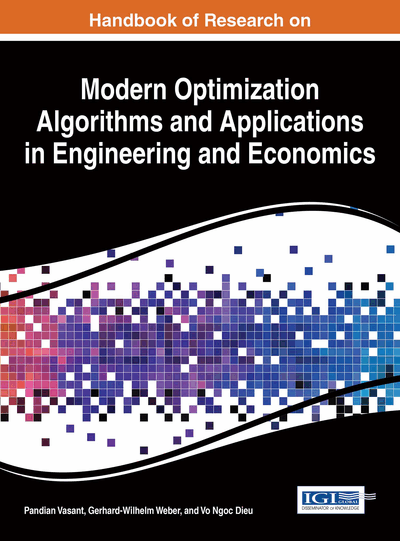# Innovative Hierarchical Fuzzy Logic for Modelling Using Evolutionary Algorithms

M. Mohammadian (University of Canberra, Australia) and R. J. Stonier (University of Canberra, Australia)
DOI: 10.4018/978-1-4666-9644-0.ch029
Available
\$37.50
No Current Special Offers

## Abstract

This paper considers issues in the design and construction of a fuzzy logic system to model complex (nonlinear) systems. Several important applications are considered and methods for the decomposition of complex systems into hierarchical and multi-layered fuzzy logic sub-systems are proposed. The learning of fuzzy rules and internal parameters is performed using evolutionary computing. The proposed method using decomposition and conversion of systems into hierarchical and multi-layered fuzzy logic sub-systems reduces greatly the number of fuzzy rules to be defined and improves the learning speed for such systems. However such decomposition is not unique and may give rise to variables with no physical significance. This can raise then major difficulties in obtaining a complete class of rules from experts even when the number of variables is small. Application areas considered are: the prediction of interest rate, hierarchical control of the inverted pendulum, robot control, feedback boundary control for a distributed optimal control system and image processing.
Chapter Preview
Top

## 1. Introduction

Conventional modelling approaches have been to construct a mathematical model and examine the system’s evolution or its control. Such approaches fail are not completely successful for many of the very large non-linear and complex systems such as for example robotic systems with large input-output data. These systems have typically high dimensionality and have degrees of uncertainty in many parameters. Progress in solving these problems has been with the aid of new advanced high-speed computers and the application of artificial intelligence paradigms, particularly neural networks and fuzzy logic for nonlinear modelling and evolutionary algorithms for optimisation methods. Application of fuzzy logic in particular, to model and solve industrial problems is now wide spread and has universal acceptance.

Fuzzy logic systems typically have a knowledge base consisting of a set of rules of the form:

where, k=1,…,n are normalised fuzzy sets for n input variables xk, k=1,…,n, and where, k=1,…,m are normalised fuzzy sets for m output variables zk, k=1,…,m. The heart of the fuzzy logic system is the inference engine that applies principles of intelligent human reasoning to interpret the rules to output an action from inputs. There are many types of inference engines in the literature, including the popular Mamdani inference engine (Wang, 1997).

Given a fuzzy rule base with M rules and n antecedent variables, a fuzzy controller as given in Equation 1 uses a singleton fuzzifier, Mamdani product inference engine and centre average defuzzifier to determine output variables, has the general form for a single output variable, say zk:

(1) whereare centres of the output setsand membership function μ defines for each fuzzy setthe value of xi in the fuzzy set, namely,. Common shapes of the membership function are typically, triangular, trapezoidal and Gaussian. A first step in the construction of a fuzzy logic system is to determine which variables are fundamentally important. Any number of these decision variables may appear, but the more that are used, the larger the rule set that must be found. It is known (Raju, Zhou & Kisner, 1990), that the total number of rules in a system is an exponential function of the number of system variables. This curse of dimensionality can be handled in a variety of ways:

## Complete Chapter List

Search this Book:
Reset